# Lecture 5 and 6 Vector calculus Integration II

• Slides: 26
Download presentation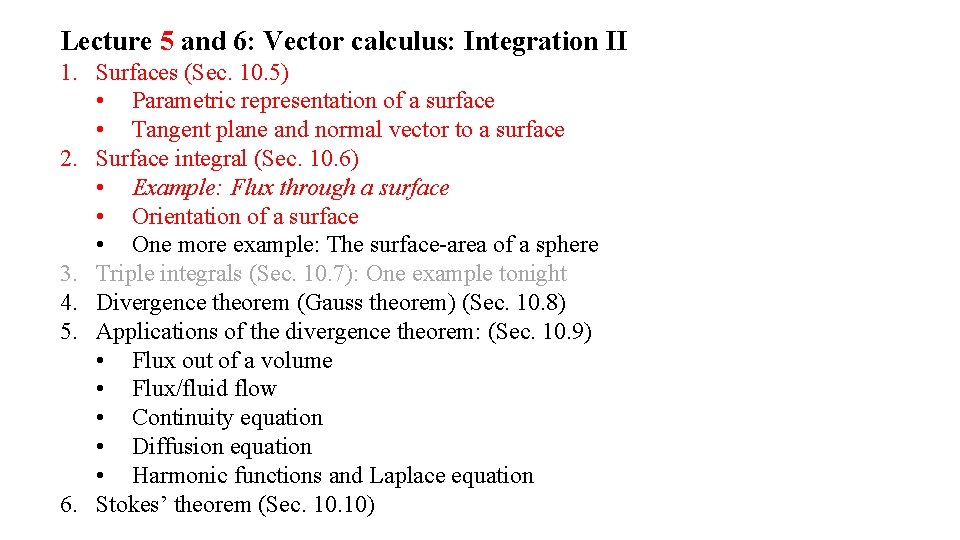Lecture 5 and 6: Vector calculus: Integration II 1. Surfaces (Sec. 10. 5) • Parametric representation of a surface • Tangent plane and normal vector to a surface 2. Surface integral (Sec. 10. 6) • Example: Flux through a surface • Orientation of a surface • One more example: The surface-area of a sphere 3. Triple integrals (Sec. 10. 7): One example tonight 4. Divergence theorem (Gauss theorem) (Sec. 10. 8) 5. Applications of the divergence theorem: (Sec. 10. 9) • Flux out of a volume • Flux/fluid flow • Continuity equation • Diffusion equation • Harmonic functions and Laplace equation 6. Stokes’ theorem (Sec. 10)Surfaces: OrientationNon-orientable surfaces: The Möbius strip and the Klein bottle The Möbius strip The Klein bottleSurface integrals, an example: Surface-area of a sphereDivergence theorem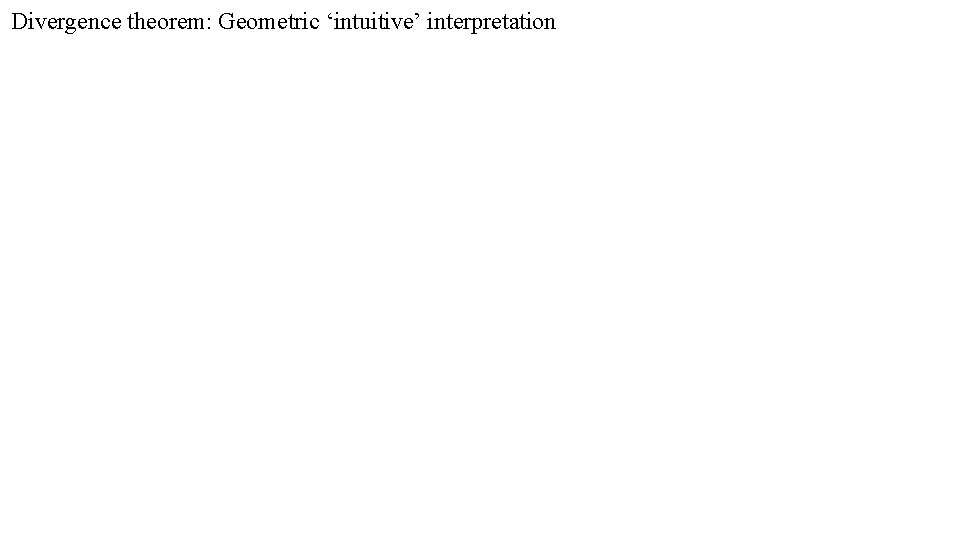Divergence theorem: Geometric ‘intuitive’ interpretationDivergence theorem: Proof, the LHS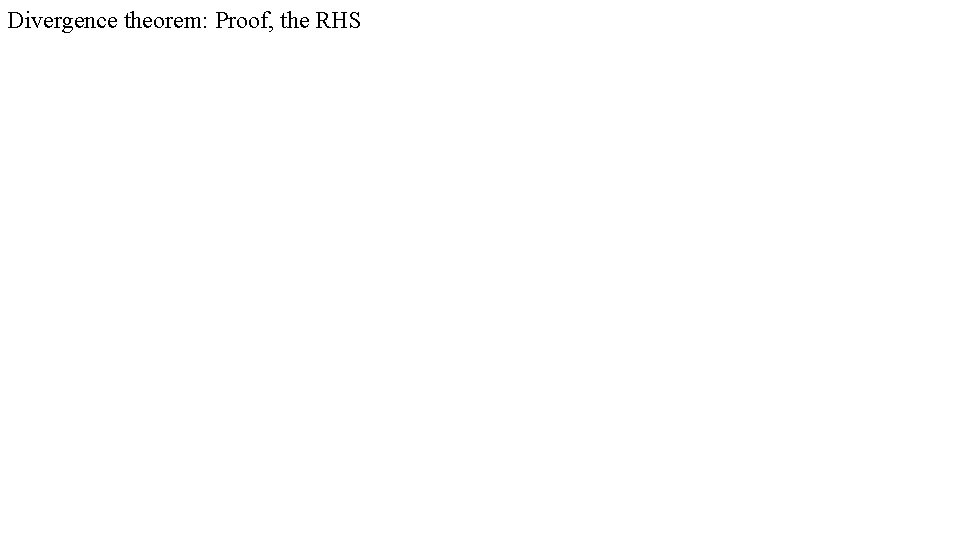Divergence theorem: Proof, the RHS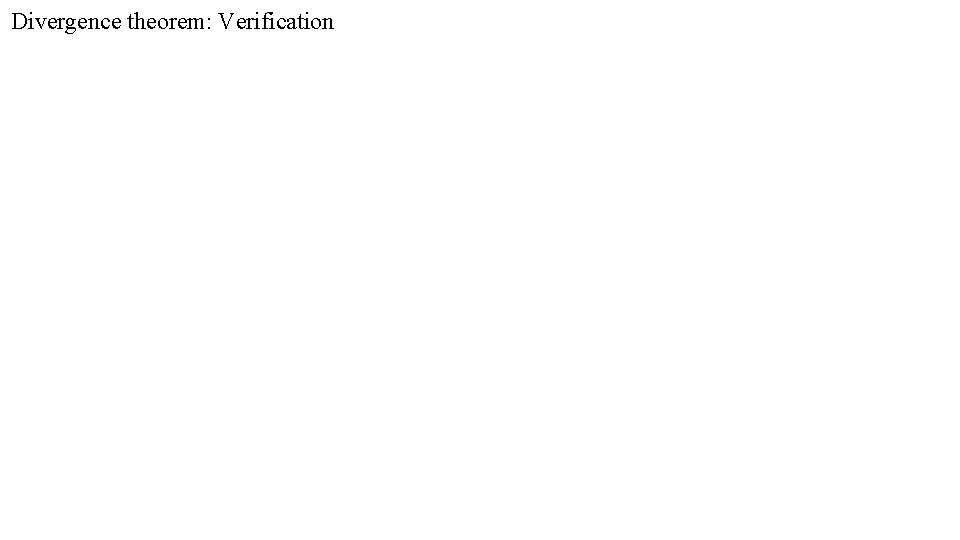Divergence theorem: VerificationDivergence theorem: VerificationDivergence theorem: VerificationSome more useful trigonometric integrals…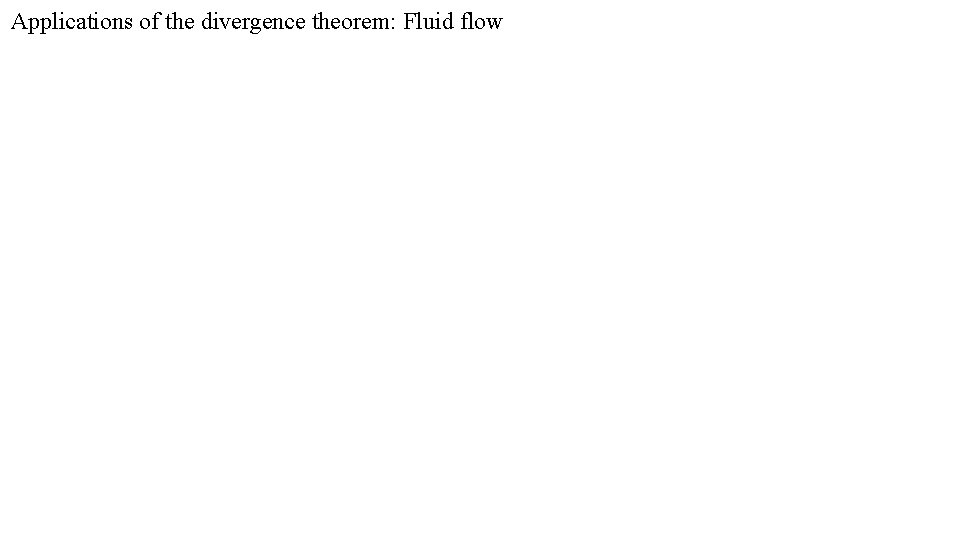Applications of the divergence theorem: Fluid flow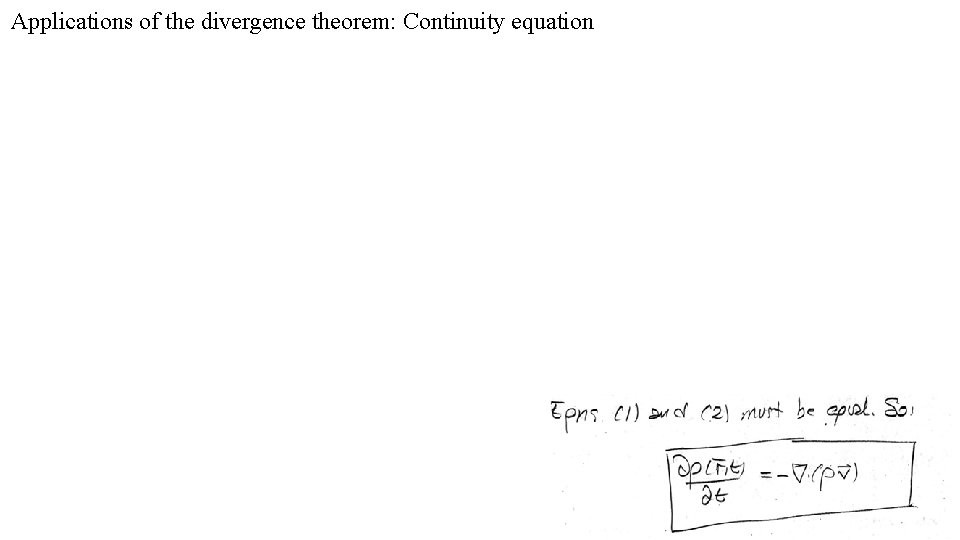Applications of the divergence theorem: Continuity equation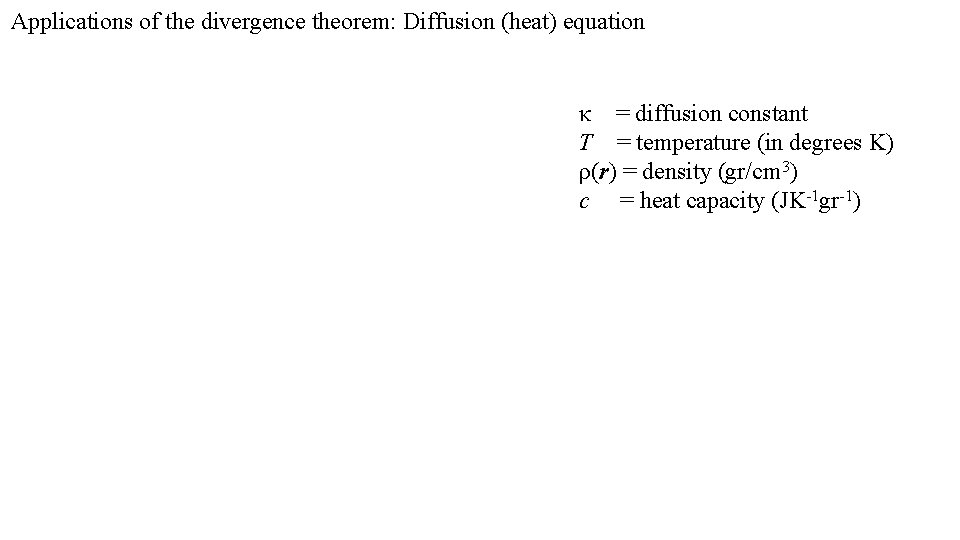Applications of the divergence theorem: Diffusion (heat) equation κ = diffusion constant T = temperature (in degrees K) ρ(r) = density (gr/cm 3) c = heat capacity (JK-1 gr-1)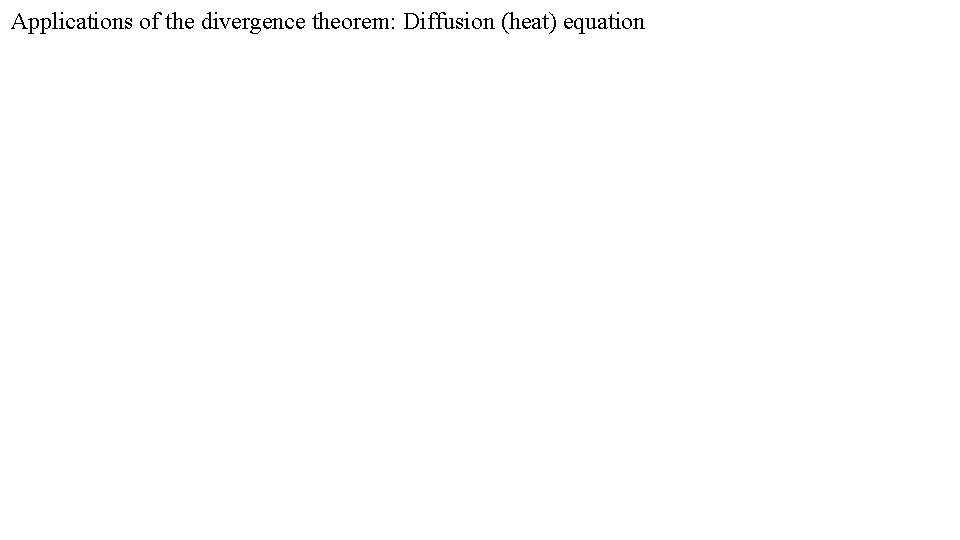Applications of the divergence theorem: Diffusion (heat) equationApplications of the divergence theorem: Diffusion (heat) equation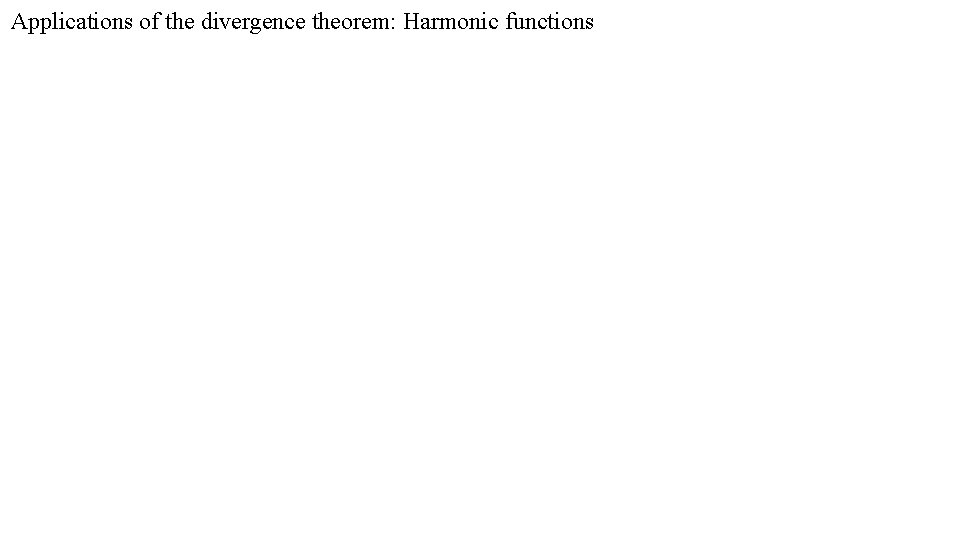Applications of the divergence theorem: Harmonic functionsHarmonic functions and Gauss’ lawApplications of the divergence theorem: Green’s theoremStokes’ theorem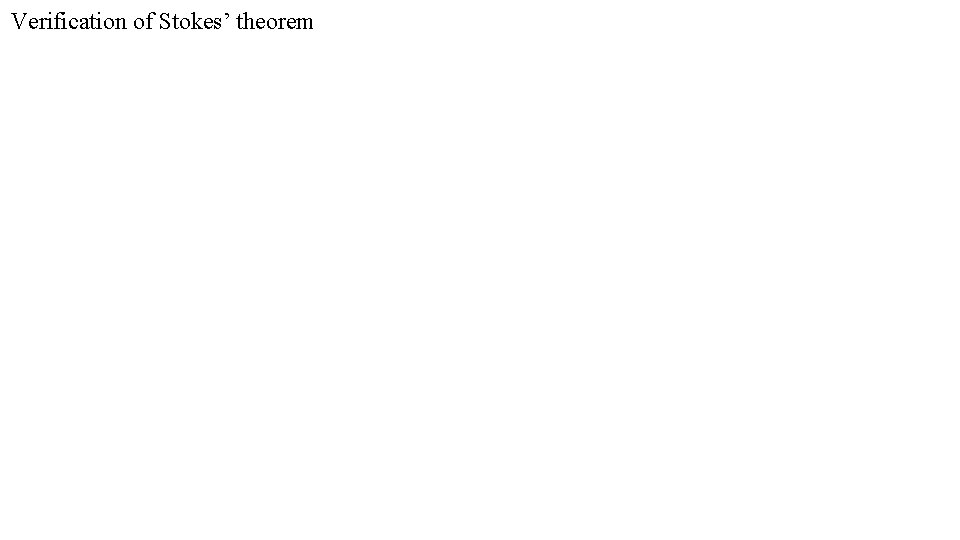Verification of Stokes’ theorem bVerification of Stokes’ theoremDuplicate slidesHarmonic functionsApplications of the divergence theorem: Diffusion (heat) equation